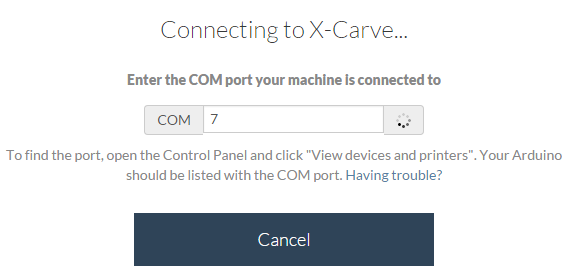# Cannot get my X-Carve started!

I can look back in this thread and see how to do the reflashing.

Not sure what you mean by board and port.

Under the tools menu you need to tell the Arduino IDE which processor you are using and what port it is on.

So, under “board” you would select Arduino Uno (or equivalent) and under the port you would select which port the Arduino is on (COM3 or whatever it is on your machine).

yup, i see it. sorry. Arduino Uno and my COM port are selected

Ok, go ahead and re-flash the Arduino with version 0.9j of grbl.

crap. 150 posts and i can’t find how to re-flash it

Try this, I’m curious if it works on Windows 8 anyway. Just use the .hex file included in the .zip

When you checked the serial monitor, was the arduino running already?

nope, didn’t work. kept telling me that the batch upload could not be completed

yessir it was

@NicShimala

Ok, do this then.

Here

ok, done

Can you now get the version number in console window when you start up the Serial Monitor?

this is what pops up

Grbl 0.9j [’\$’ for help]

Great. Enter the \$\$ command and post the results here.

Grbl 0.9j [’\$’ for help]
\$0=10 (step pulse, usec)
\$1=255 (step idle delay, msec)
\$4=0 (step enable invert, bool)
\$5=0 (limit pins invert, bool)
\$6=0 (probe pin invert, bool)
\$11=0.020 (junction deviation, mm)
\$12=0.002 (arc tolerance, mm)
\$13=0 (report inches, bool)
\$20=0 (soft limits, bool)
\$21=0 (hard limits, bool)
\$22=0 (homing cycle, bool)
\$24=25.000 (homing feed, mm/min)
\$25=750.000 (homing seek, mm/min)
\$26=250 (homing debounce, msec)
\$27=1.000 (homing pull-off, mm)
\$100=40.000 (x, step/mm)
\$101=40.000 (y, step/mm)
\$102=320.000 (z, step/mm)
\$110=8000.000 (x max rate, mm/min)
\$111=8000.000 (y max rate, mm/min)
\$112=500.000 (z max rate, mm/min)
\$120=500.000 (x accel, mm/sec^2)
\$121=500.000 (y accel, mm/sec^2)
\$122=50.000 (z accel, mm/sec^2)
\$130=290.000 (x max travel, mm)
\$131=290.000 (y max travel, mm)
\$132=100.000 (z max travel, mm)
ok

Ok, things are looking pretty good.

If you have the ACME screw change \$102 to 188.976
\$102=188.976

i have the M8. didn’t upgrade that yet

Now try to run the Machine Setup in Easel.

this is all i’m getting…Check the version number of Easel Local.